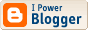## Why Health Guidelines are Unreliable

Caution: This posting contains mathematical expressions that may cause stress.

Today's New York Times Magazine has an article by Gary Taubes pointing out the limitations of epidemiology in telling people what is good for them. I found the article far too long for its content and also taking rather a narrow view of the topic. Taubes has just written a book about diets, "Good Calories, Bad Calories", but it is not out yet (publication date is Sept. 25) and therefore I have no idea whether he deals with the issue of this blog.

My point here is that a major limitation of health guidelines is the lack of scientific foundations behind them.

Let us consider the example of weight. There is the infamous body mass index (BMI) that it supposed to have an optimal value around 25. To find your BMI you take your weight in kilograms and divide it by the square of your height in meters. (You can tell right away the European origin of the measure. For the conversion you need to multiply pounds by 2.2 to get kilograms and inches by 0.254 to get meters.) You can of course express your ideal weight in kilograms from the formula below, provided the height is expressed in meters.

Ideal Weight = 25 * height2

Let us now look at the results for people 1.60m (5 feet 3 inches), 1.70m (5 feet 7 inches), 1.80m (5 feet 11 inches), 1.90m (6 feet 2 inches) . The weights (in kilograms) we get from the formulas are respectively: 64, 72, 81, and 90. Do you notice that the weight comes to be close to the number expressed by the decimals in your height? When I was growing up in Greece doctors were saying that your ideal weight should be in kilograms to the number expressed by the decimals in your height. The mathematical formula for that is a linear equation

Old Ideal Weight = 100 * (height - 1)

That apparently was an old heuristic and the new "scientific" guidelines wanted to be close to it. The straight line of the linear equation is a tangent to the parabola of the quadratic equation and the two meet for height equal to 2 meters and weight equal to 100 kgs. However they are very close over a large area. For height 1.80m the difference of the equations is only 1kg and for height 1.70m is 2.25kg. I suspect the old formula was derived as a gross approximation to a set of observed weights and heights, most likely from recruits in a Western European army. (I have no evidence for that but data from army recruits have been used often in population studies because they were readily available.)

However a linear formula is itself too coarse an approximation for a large range of heights (if we want to include, for example women and children and not only young men) so the quadratic formula given first was adopted. Of course, the new formula had to agree with the old for the heights common to young men, hence the coefficient value of 25.

But there is another disturbing fact in that formula. Why is the weight expressed as the square of the height? Elementary solid geometry tells us that the volume of an object is equal to the third power of its linear dimensions, so the weight should be proportional of the cube of the height. However strength is proportional to the cross section of your arms and legs, so it is proportional to the square of the height. As a result, the ratio of strength over volume is declining function of height and that is why insects are far more mobile than dogs and dogs are more mobile than humans. Because for any given person height is determined by genetics and childhood nutrition, it must be taken as a given. Then the formula for proper weight must contain both a cubic term (to account for the volume) and a quadratic term (to account for the need for strength). The formula for the BMI is certainly flawed but nobody has figured out the right formula.

Not surprisingly, there have been articles pointing out that it gives too low weights for tall people but the public is not aware of them. Another flaw of the formula (also recognized in the literature) is that it does not distinguish between weight due to muscle and weight due to fat. Again that knowledge is not widely available.

Yes, you must be concerned about gaining excess weight, but what is excess weight is not what the formula tells you. Your best bet is to find a health practitioner who takes a realistic view of weight and discuss the problem with that person.

Labels: , , ,